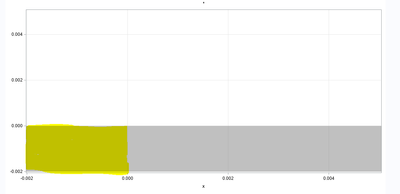## Boundary for band in SGPLOT

Hello SAS Community,

I use band to annotate graph with gray box. But I need box to finish at x=0, and it keep going. How I stop the grey box at 0, just get the yellow area (below)?I use code:

``````
data boundary;
do x = -0.002 to 0 by .00001;
up1=0;
output;
end;
run;

data plot;
set plot_data boundary;
run;

proc sgplot data=plot;
band x=x upper=0 lower=-0.002 / fillattrs=(transparency=0.5 color=gray);
yaxis max = 0.005 grid;
xaxis max = 0.005 grid;
run;``````

Thank you for help!

## Re: Boundary for band in SGPLOT

Hello @linlin87,

I think the issue is that you specify a constant value in the UPPER= option of the BAND statement rather than the variable UP1, which you defined in the BOUNDARY dataset. So try this:

`band x=x upper=up1 lower=-0.002 / fillattrs=(transparency=0.5 color=gray);`

Depending on the content of dataset PLOT_DATA and how you use it in your real PROC SGPLOT step, other techniques to limit the gray box include:

• the NOEXTEND option of the BAND statement
• defining a step function in the BOUNDARY dataset:
```data boundary;
do x = -0.002 to 0.005 by .0001;
x=round(x,1e-9);
if x<=0 then up1=0;
else up1=-0.002;
output;
end;
run;```

If the box doesn't look exactly rectangular, you may also want to add the TYPE=STEP option to the BAND statement.

From SAS Users blog